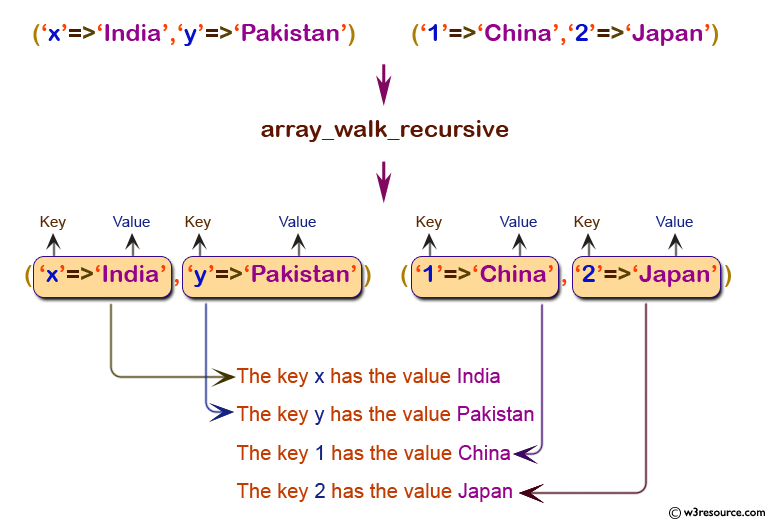# PHP : array_walk_recursive() function

## PHP: Apply a user function recursively to every member of an array

The array_walk_recursive() function apply a user defined function to every element of an array recursively. The user-defined function takes array's values and keys as parameters.

Version:

(PHP 5 and above)

Syntax:

`array_walk_recursive(input_array, user_function, user_data)`

Parameters:

 Name Description Required / Optional Type input_array The input array. Required Array user_function The name of the user-defined function. Required user_data Additional parameter. If it is supplied, it will be passed as the third parameter to the user_function. Optional Mixed*

Return value:

TRUE on success or FALSE on failure.

Value Type: Boolean

Example:

``````<?php
function w3rfunction(\$value,\$key)
{
echo "The key \$key has the value \$value<br />";
}
\$tutorial1=array("x"=>"india","y"=>"Pakistan");
\$tutorial2=array(\$tutorial1,"1"=>"China","2"=>"Japan");
array_walk_recursive(\$tutorial2,"w3rfunction");
?>
``````

Output:

```The key x has the value india
The key y has the value Pakistan
The   key 1 has the value China
The key 2 has the value Japan ```

Pictorial Presentation:View the example in the browser

Practice here online :

PHP Function Reference

Previous: array_values
Next: array_walk

﻿

## PHP: Tips of the Day

PHP: Extract numbers from a string

```\$str = 'In My Cart : 11 12 items';
preg_match_all('!\d+!', \$str, \$matches);
print_r(\$matches);
```